Next: Cross-check with other GRB Up: Automatic Informations Previous: Automatic Informations   Contents

#### The Visibility Parameter

The line reporting the Sun Visib.'', which stands for Sun Visibility'', has the following meaning: the visibility of a given direction from the BeppoSAX line of sight, is defined as follows: it is set to 0, when it is hidden by the Earth, otherwise it is 2 when it is visible; in the case of extended sources, like the Sun, whose apparent angular diameter is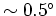, the visibility can assume two other values, when only a fraction of the source is Earth-blocked: -1, when the center is occulted, and 1, when this is visible. This example shows a null visibility, therefore the spacecraft was on the dark side of the Earth, i.e. at night, at the burst trigger time: this info turns to be very useful, whenever it is important to establish whether the burst candidate might have come from the Sun.

Another important piece of information that is extracted from the satellite ephemeris is the direction of the Earth center, as seen from the BeppoSAX frame of reference: its local coordinates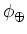and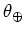are calculated at the burst time and reported within the line, expressing Earth Phi'' and Theta''.

Then, the next two lines have the following meanings: the first reports the values of the four LS E-elev'' parameters: these express the elevation angles of the four GRBM units above the Earth limb; in other words, when the elevation angle is positive, it means that the normal direction to the corresponding GRBM unit points to the sky, otherwise to some point of the Earth surface below the satellite. The second line reports the same informations, but through different parameters: actually, the LS Ecos'' express the four cosines of the angles between the normal directions to the four GRBM units and the Earth center direction, respectively (this choice was driven by some needs connected with the GRBM localization procedure).

Finally, the last line reports the following parameter: GRBM-AC angle (deg)'': this is computed as follows: let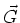be a four-dimensional vector, whose components are the four fluences (or total counts) in the four GRBM units, in the 40-700 keV (GRBM) band; similarly, let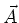be the corresponding four-dimensional vector for the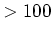keV (AC) band; the GRBM-AC angle is defined by the eq.: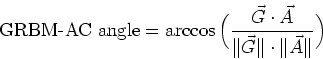(31)

This parameter was originally conceived to evaluate how similarly the total counts divide among the four GRBM units between the two cases, i.e. for the GRBM and AC energy bands. This angle, which is always positive, as it trivially follows from its definition, has the following property: the smaller this angle, the more similar the ratios between the corresponding units in the two energy bands. In other words, this angle allows to test how similar the hardness ratios of different detector units are, therefore it verifies the same property as the HRR parameter does (see sec.), only in a different way. To some extent, this parameter has come out be a bit redundant: therefore, hereafter it will be taken into account no longer.Next: Cross-check with other GRB Up: Automatic Informations Previous: Automatic Informations   Contents
Cristiano Guidorzi 2003-07-31